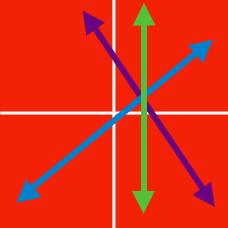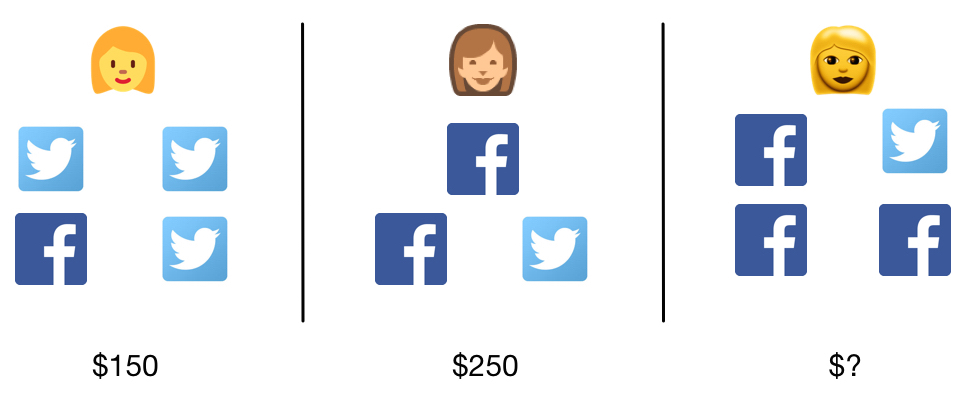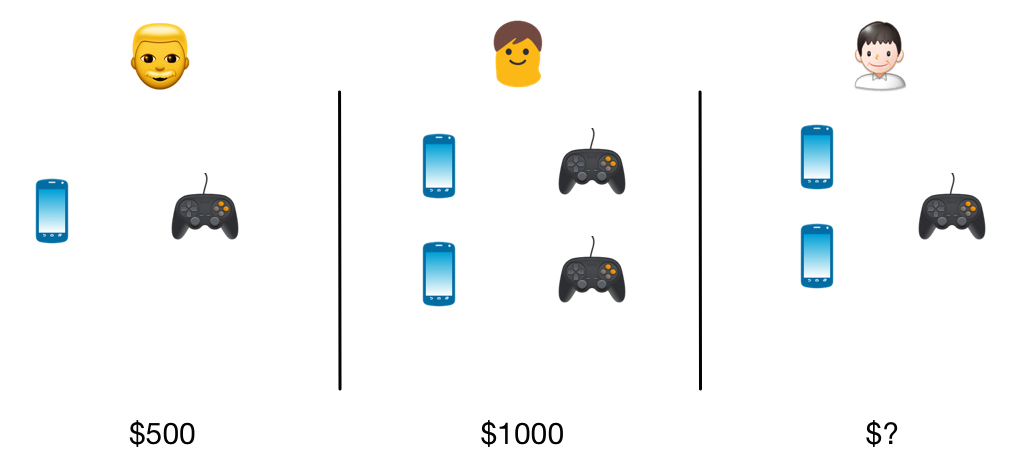Algebra

System of Linear Equations - Substitution

$x$ and $y$ are real numbers that satisfy $x = 7$ and $x + y = 9$. What is the value of $y$?

$x$ and $y$ are real numbers that satisfy $2x = 50$ and $x + y = 40$. What is the value of $y$?Alice has 1 Facebook stock and 3 Twitter stocks, and her portfolio is worth $150. Brenda has 2 Facebook stocks and 1 Twitter stock, and her portfolio is worth$250.
Candice has 3 Facebook stocks and 1 Twitter stock. How much is her portfolio worth?On Black Friday,
Joel bought 1 phone and 1 PS4 for $500, and Kelvin bought 2 phones and 2 PS4's for$1000.
Linus bought 2 phones and 1 PS4.

Can we determine how much Linus paid?

$x$ and $y$ are real numbers that satisfy $5x -8 = 7$ and $3x - 2y = - 9$. What is the value of $y$?

×